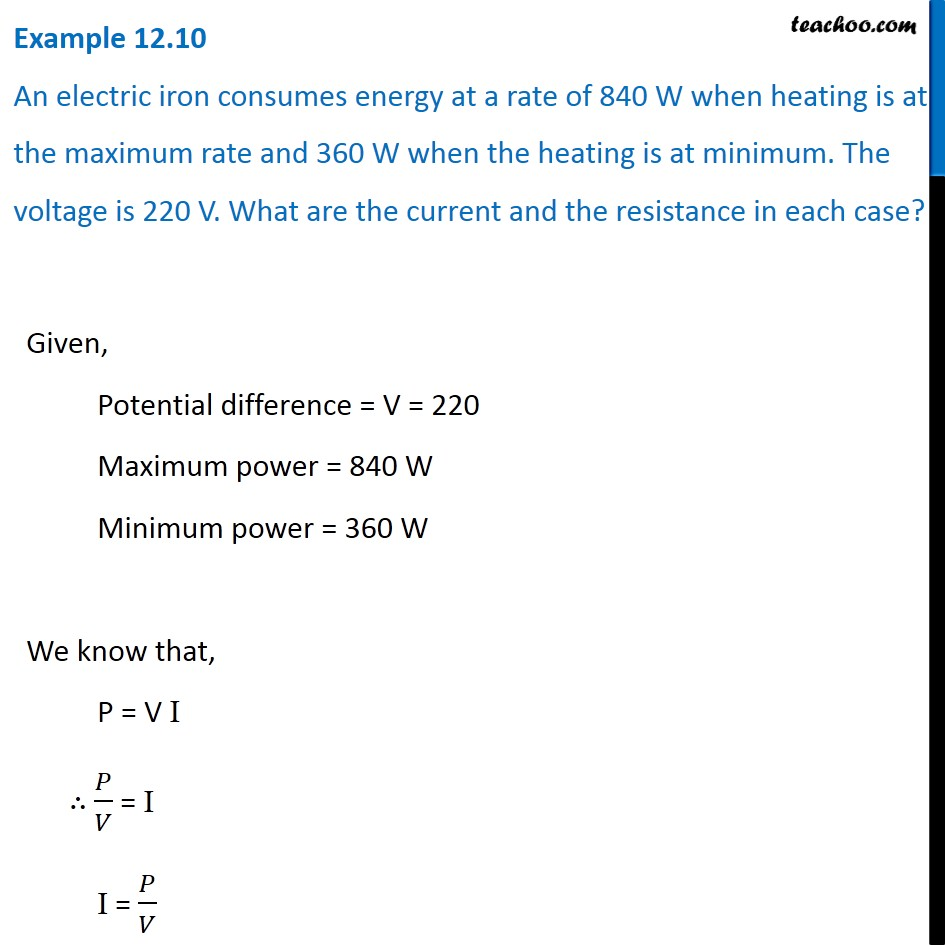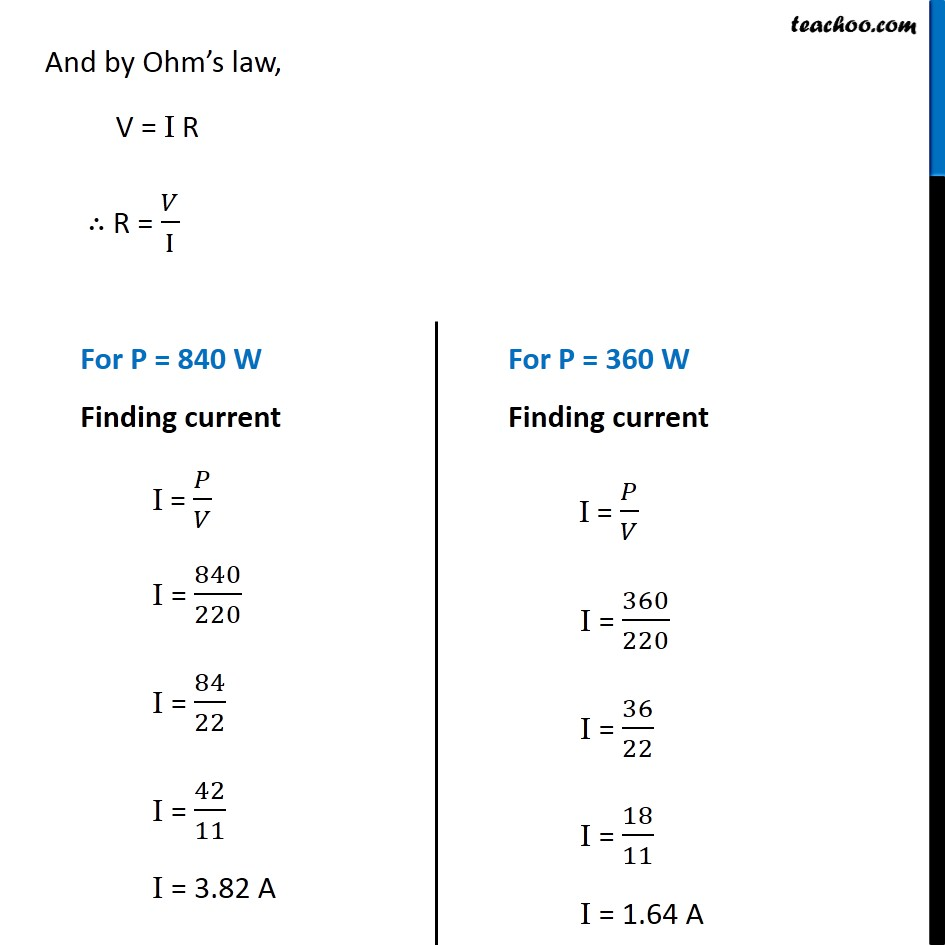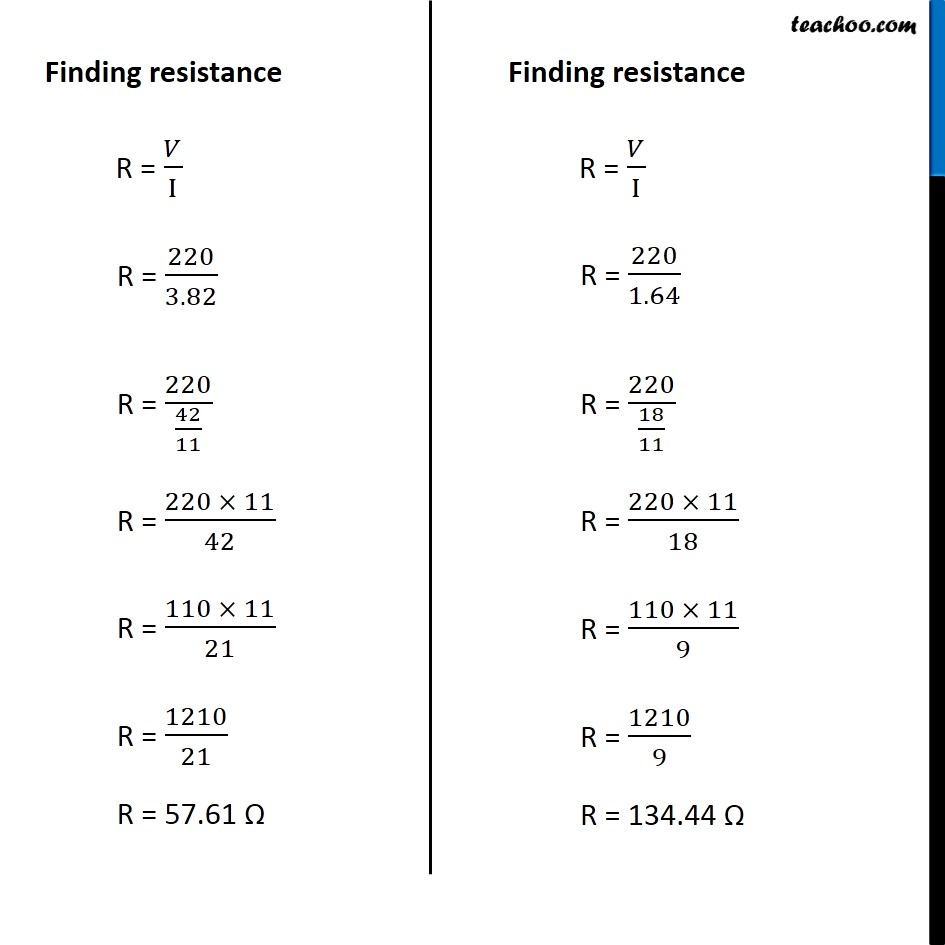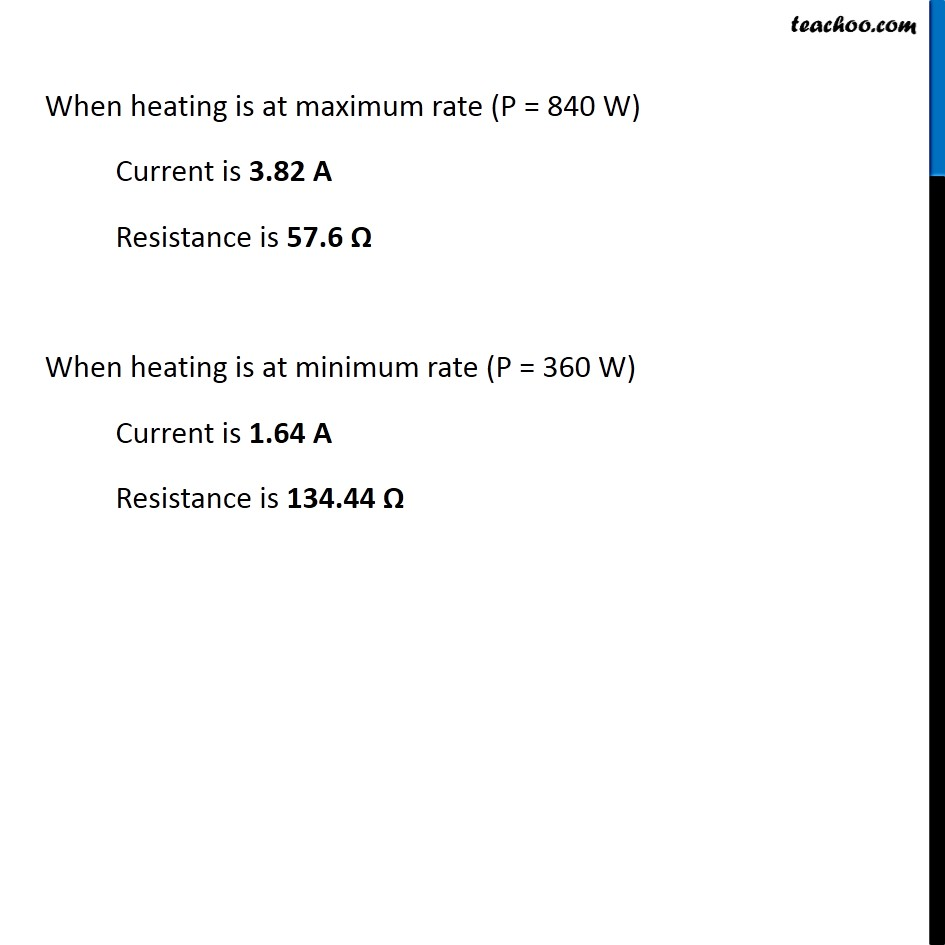Examples from NCERT Book

Class 10
Chapter 12 Class 10 - Electricity

## An electric iron consumes energy at a rate of 840 W when heating is at the maximum rate and 360 W when the heating is at minimum. The voltage is 220 V. What are the current and the resistance in each case?Learn in your speed, with individual attention - Teachoo Maths 1-on-1 Class

### Transcript

Example 12.10 An electric iron consumes energy at a rate of 840 W when heating is at the maximum rate and 360 W when the heating is at minimum. The voltage is 220 V. What are the current and the resistance in each case? Given, Potential difference = V = 220 Maximum power = 840 W Minimum power = 360 W We know that, P = V I ∴ 𝑃/𝑉 = I I = 𝑃/𝑉 And by Ohm’s law, V = I R ∴ R = 𝑉/I For P = 840 W Finding current I = 𝑃/𝑉 I = 840/220 I = 84/22 I = 42/11 I = 3.82 A For P = 360 W Finding current I = 𝑃/𝑉 I = 360/220 I = 36/22 I = 18/11 I = 1.64 A Finding resistance R = 𝑉/I R = 220/3.82 R = 220/(42/11) R = (220 × 11)/42 R = (110 × 11)/21 R = 1210/21 R = 57.61 Ω Finding resistance R = 𝑉/I R = 220/1.64 R = 220/(18/11) R = (220 × 11)/18 R = (110 × 11)/9 R = 1210/9 R = 134.44 Ω When heating is at maximum rate (P = 840 W) Current is 3.82 A Resistance is 57.6 Ω When heating is at minimum rate (P = 360 W) Current is 1.64 A Resistance is 134.44 Ω